Courses

# Test: Properties of Gases - 1

## 10 Questions MCQ Test Topicwise Question Bank for Mechanical Engineering | Test: Properties of Gases - 1

Description
This mock test of Test: Properties of Gases - 1 for Mechanical Engineering helps you for every Mechanical Engineering entrance exam. This contains 10 Multiple Choice Questions for Mechanical Engineering Test: Properties of Gases - 1 (mcq) to study with solutions a complete question bank. The solved questions answers in this Test: Properties of Gases - 1 quiz give you a good mix of easy questions and tough questions. Mechanical Engineering students definitely take this Test: Properties of Gases - 1 exercise for a better result in the exam. You can find other Test: Properties of Gases - 1 extra questions, long questions & short questions for Mechanical Engineering on EduRev as well by searching above.
QUESTION: 1

Solution:
QUESTION: 2

Solution:
QUESTION: 3

### All of the following statements are correct, except

Solution:
QUESTION: 4

If value of n is infinitely large in a polytropic process P Vn= C, then the process is known as constant

Solution: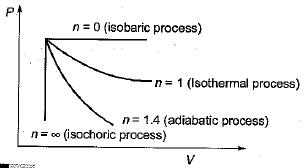QUESTION: 5

Match List-I (Isotherms) with List-ll (Value of T/TC) pertaining to the generalized compressibility charts in terms of reduced properties and select the correct answer.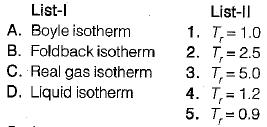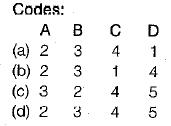Solution:
QUESTION: 6

In the Van der Waal’s equation, the unit of constant a is

Solution: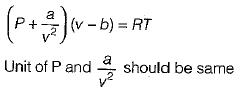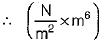is unit of a, so unit of a is Nm4

QUESTION: 7

The temperature at which a real gas obeys the ideal gas laws over a wide range of pressure is called

Solution:
QUESTION: 8

For an ideal gas, the slope of the constant- volume line in the T-S diagram is

Solution:QUESTION: 9

The work done in a reversible adiabatic process from state 1 to state 2 is given by

Solution:
QUESTION: 10

Absolute zero pressure will occur

Solution: